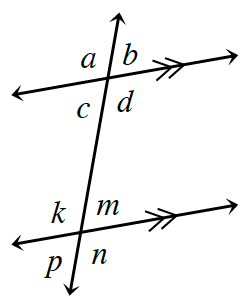### Home > GC > Chapter 8 > Lesson 8.3.2 > Problem8-98

8-98.Find the missing angle(s) in each problem below using the geometric relationships shown in the diagram at right. Be sure to write down the conjecture that justifies each calculation. Remember that each part is a separate problem.

1. If $d=110°$ and $k = 5x - 20°$, write an equation and solve for $x$.

Note that $\angle d$ and $\angle k$ are alternate interior angles.
What is true about alternate interior angles?

2. If $b=4x−11°$ and $n=x+26°$, write an equation and solve for $x$. Then find the measure of $\angle n$.

Note that $\angle b$ is equal to $\angle m$ and that $\angle m$ and $\angle n$ are supplementary angles.
Refer to the Math Notes box in Lesson 2.1.4 if you need help justifying why.